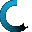Retrieving data ...
Please do not click anywhere else.

If you see no response after 2-3 minutes, then click on the [X] button in the upper right corner to close the window and retry the operation at a later time.
Interactive Multimedia Learning & Teaching Management System Suite## Course Contents

Course Name: 5th Grade Math (New)
• 1. Basic Numbers
•  1.1 Place Values and Expanded Form
•  1.2 Whole Numbers
•  1.3 Decimal Numbers
•  1.4 Prime Numbers
•  1.5 Composite Numbers
•  1.6 The Number Line
•  1.7 Positive and Negative Integers
•  1.8 Real Numbers
•  1.9 Absolute Value
•  1.10 Rounding and Estimating
• 2. Basic Number Operations Review
•  2.1.1 Addition with 3-Digit Numbers
•  2.1.2 Addition with 4-Digit Numbers
•  2.1.3 Addition with 5-Digit Numbers
•  2.1.4 Addition with 6-Digit Numbers
•  2.1.5 Addition with 7-Digit Numbers
•  2.1.7 Adding Three Sets of Numbers
•  2.1.9 Addition of Whole Numbers and Decimals
•  2.1.10 Addition with Negative Numbers
•  2.2 Subtraction
•  2.2.1 Subtraction with 3-Digit Numbers
•  2.2.2 Subtraction with 4-Digit Numbers
•  2.2.3 Subtraction with 5-Digit Numbers
•  2.2.4 Subtraction with 6-Digit Numbers
•  2.2.5 Subtraction with Equations
•  2.2.6 Subtraction with Decimals
•  2.2.7 Subtraction Involving Whole Numbers and Decimals
•  2.2.8 Subtraction with Negative Numbers
•  2.3 Multiplication
•  2.3.1 Multiplying a 2-Digit Number by a 1-Digit Number
•  2.3.2 Multiplying a 3-Digit Number by a 1-Digit Number
•  2.3.3 Multiplying a 4-Digit Number by a 1-Digit Number
•  2.3.4 Multiplying a 2-Digit Number by a 2 Digit Number
•  2.3.5 Multiplying a 3-Digit Number by a 2-Digit Number
•  2.3.6 Multiplying a 4-Digit Number by a 2-Digit Number
•  2.3.7 Multiplying Whole Numbers and Decimal Numbers
•  2.3.8 Multiplying Two Decimal Numbers
•  2.3.9 Multiplication with Equations
•  2.4 Division
•  2.4.1 Understanding Division and Division Terms
•  2.4.2 Division without a Remainder
•  2.4.3 Division with a Remainder
•  2.4.4 Dividing a 3-Digit Number by a 2-Digit Number
•  2.4.5 Dividing a 4-Digit Number by a 2-Digit Number
•  2.4.6 Dividing a 4-Digit Number by a 3-Digit Number
•  2.4.7 Dividing a 5-Digit Number by a 2-Digit Number
•  2.4.8 Dividing a 5-Digit Number by a 3-Digit Number
•  2.4.9 Division with Decimal Numbers
•  2.4.10 Dividing Two Decimal Numbers
• 3. Fractions
•  3.1 Understanding Fractions
•  3.2 Equivalent Fractions
•  3.3 The Greatest Common Factor
•  3.4 The Least Common Multiple
•  3.5 The Least Common Denominator
•  3.6 Mixed Fractions
•  3.7 Converting Mixed Fractions to Improper Fractions
•  3.8 Converting Improper Fractions to Mixed Fractions
• 4. Operations with Fractions
•  4.1 Reducing Fractions
•  4.3 Adding Fractions and Whole Numbers
•  4.5 Multiplying Fractions
•  4.6 Multiplying Fractions and Whole Numbers
•  4.7 Subtracting Fractions
•  4.8 Subtracting Fractions and Whole Numbers
•  4.9 Subtracting Mixed Fractions
•  4.10 Dividing Fractions
•  4.11 Dividing Fractions and Whole Numbers
•  4.12 Converting Fractions into Decimal Numbers
•  4.13 Converting Decimal Numbers into Fractions
• 5. Number Comparison
•  5.1 The Number Line
•  5.2 Comparing Whole Numbers
•  5.3 Ordering Whole Numbers
•  5.4 Comparing Decimal Numbers
•  5.5 Ordering Decimal Numbers
•  5.6 Comparing Fractions
•  5.7 Ordering Fractions
•  5.8 Comparing Mixed Fractions
•  5.9 Comparing Whole Numbers and Fractions
• 6. Percentages
•  6.1 Introduction to Percentages
•  6.2 Converting a Percent into a Fraction
•  6.3 Converting a Percent into a Decimal Number
•  6.4 Converting a Decimal Number into a Percent
•  6.5 Converting a Fraction into a Percent
•  6.6 Finding the Percentage of a Number
•  6.7 Determining the Percentage When Given Two Numbers
• 7. Math in Everyday Life
•  7.1 Salary Calculation
•  7.2 Number Games
•  7.3 Money Games
•  7.4 Family Budgeting
•  7.5 Age Comparisons
•  7.6 Calculating Commission
•  7.7 Calculating Sale Price
•  7.8 Interest Rates
• 8. Measurements and Conversions
•  8.1 Time
•  8.2 Systems of Measurement
•  8.3 Mass and Weight
•  8.4 Length
•  8.5 Temperature
•  8.6 Volume
•  8.7 Rate, Time and Distance Traveled
• 9. Geometry - Angles
•  9.1 Angles
•  9.2 Measuring Angles
•  9.3 Types of Angles
• 10. Geometry - Basic Shapes and Solids
•  10.1 Triangles
•  10.3 Rectangles and Rhombus
•  10.4 Polygons
•  10.5 Circles
•  10.6 Cubes
•  10.7 Pyramids
•  10.8 Cylinders
•  10.9 Rectangular Prisms
• 11. Geometry - Working with Shapes and Solids
•  11.1 Perimeter and Area of Triangles
•  11.2 Perimeter and Area of Squares and Rectangles
•  11.3 Perimeter and Area of Parallelograms and Trapezoids
•  11.4 Circumference and Area of Circles
•  11.5 Surface Area and Volume of Cubes
•  11.6 Surface Area and Volume of Rectangular Prisms
• 12. Algebra - Variables, Expressions and Equations
•  12.1 Variables
•  12.2 Expressions and Equations
•  12.3 Writing Expressions and Equations
•  12.4 Word Problems
• 13. Algebraic Operations
•  13.1 Like and Unlike Terms
•  13.2 The Addition Operation with Variables
•  13.3 The Subtraction Operation with Variables
•  13.4 The Multiplication Operation with Variables
•  13.5 The Division Operation with Variables
•  13.6 Canceling Variables
•  13.7 Evaluating Expressions with Variables
• 14. Algebra - The Coordinate Plane and First Quadrant
•  14.1 Introduction to the Coordinate Plane
•  14.2 Working with First Quadrant
•  14.3 Connecting Points in the First Quadrant
• 15. Graphs - Collecting and Displaying Data
•  15.1 Line Graphs
•  15.2 Bar Graphs
•  15.3 Double Bar Graphs
•  15.4 Circle (Pie) Graphs
•  15.5 Surveys and Experiments
• 16. Probability
•  16.1 Introduction to Probability
•  16.2 Making Predictions with Probability